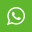## Material Content for PROGRAMMING AND PROBLEM SOLVING THROUGH C LANGUAGE July 2015

1. Each question below gives a multiple choice of answers. Choose the most appropriate one and enter in the “OMR” answer sheet supplied with the question paper, following instructions therein. (1x10)

1.1 Which of the following is the correct order of evaluation for the below expression?

z = x + y * z / 4 % 2 - 1

A) * / % + - =

B) = * / % + -

C) / * % - + =

D) * % / - + =

1.2 Which of the following is the correct order if calling functions in the below code?

a = f1(23, 14) * f2(12/4) + f3();

A) f1, f2, f3

B) f3, f2, f1

C) Order may vary from compiler to compiler

D) None of the above 1.3 How many times "IndiaBIX" is get printed?

int main()

{

int x;

for(x=-1; x<=10; x++)

{

if(x < 5)

continue;

else

break;

printf("IndiaBIX");

}

return 0;

}

A) Infinite times

B) 11 times

C) 0 time

D) 10 times

1.4 What do the following declaration signify? void *cmp();

A) cmp is a pointer to an void type.

B) cmp is a void type pointer variable.

C) cmp is a function that return a void pointer.

D) cmp function returns nothing.

1.5 When following piece of code is executed, what output will be generated?

#include

int main(){

char arr="Network";

printf("%s", arr);

return 0; }

A) Network

B) N

C) Garbage value

D) Compilation error

1.6 The result of a Relational operation is always

A) either True or False

B) is less than or is more than

C) is equal or less or more

D) All of the above

1.7 The keyword used to transfer control from a function back to the calling function is

A) switch

B) goto

C) go back

D) return

1.8 What is the similarity between a structure, union and enumeration?

A) All of them let you define new values

B) All of them let you define new data types

C) All of them let you define new pointers

D) All of them let you define new structures

1.9 Which of the following cannot be used as identifiers?

A) Spaces

B) Digits

C) Underscores

D) Letters

1.10 How many times is a do while loop guaranteed to loop?

A) 0

B) Infinitely

C) 1

D) Variable

2. Each statement below is either TRUE or FALSE. Choose the most appropriate one and ENTER in the “OMR” answer sheet supplied with the question paper, following instructions therein. (1x10)

2.1 Functions can be called either by value or reference.

2.2 Comma operator is used for separation.

2.3 The size of a structure can be determined by both size of variable name and size of (struct tag).

2.4 While loop is post tested loop.

2.5 All of the following are valid expressions in C.

a = 2 + (b = 5);

a = b = c = 5;

a = 11 % 3;

2.6 A variable is a string that varies during program execution.

2.7 void (*ptr)() is a pointer to a function which receives nothing and returns nothing.

2.8 Calling a uninitialized variable will provide zero value.

2.9 C language cannot be used for database manipulation.

2.10 In the expression a=b=5 the order of Assignment is NOT decided by Associativity of operators.

3. Match words and phrases in column X with the closest related meaning/ word(s)/phrase(s) in column Y. Enter your selection in the “OMR” answer sheet supplied with the question paper, following instructions therein. (1x10)

 question option 3.1 size of long int in byte A. pp is pointer to an array of integer 3.2 pp[i] can be written as B. Unending loop if no break statement inside the body 3.3 Union C. returning a pointer to the beginning of the block 3.4 malloc() D. pp is function that returns pointer to integer 3.5 typedef E. allocates memory but does not clear memory 3.6 int(*pp) F. two 3.7 Structure G. can be used to create variables of old types 3.8 calloc( ) H. one 3.9 int(*pp)(void*,void*) I. is a memory location that is used by several different variables, which may be of different type 3.10 while(1) J. *(d + i) K. is different memory location that is used by several different variables, which may be of different type L. can be used to create variables of new types M. allocate and clear memory

4. Each statement below has a blank space to fit one of the word(s) or phrase(s) in the list below. Enter your choice in the “OMR” answer sheet supplied with the question paper, following instructions therein. (1x10)

4.1 An array element is accessed using an ________ number.

4.2 NULL is macro constant which has been defined in the header file ________.

4.3 Function ________ repositions the file position pointer to the beginning of the file.

4.4 A library function ________ causes an exit from the program in which it occurs.

4.5 The ________ statement causes an exit from the innermost loop or switch.

4.6 ________ data member can only be used in static functions.

4.7 ________ cannot be legitimately passed to a function.

4.8 The smallest data item a computer can process is called a(n) ________.

4.9 A function call mechanism that passes arguments to a function by passing a copy of the values of the arguments is ________.

4.10 Name the header file to be included for the use of built in function isalnum() is ________.

A. Bit B. header file C. fseekf D. call by value E. static F. exit G. index H. stdio.h I. break J. Ctype.h K. unstructured L. getc M. pointer

## View MoreMaterial

### Share

#### .share-buttons{ list-style: none; } .share-buttons li{ display: inline; }